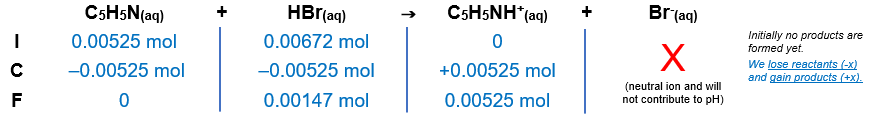# Problem: Calculate the pH for each of the following cases in the titration of 25.0 mL of 0.210 M pyridine, C5H5N(aq) with 0.210 M HBr(aq) (Kb = 1.7 x 10-9)after addition of 32.0 mL of HBr

###### FREE Expert Solution

HBr (strong acid) will react with C5H5N (weak base).

C5H5N(aq) + HBr(aq) → C5H5NH+(aq) + Br-(aq)

Step 1: Calculate the initial amount (in moles) of each species:

moles C5H5N = 0.00525 mol C5H5N

moles HBr = 0.00672 mol HBr

Step 2: Construct an ICF chart to determine the final amount of each species.After the reaction is complete the solution contains:

0.00147 mol HBr → strong acid
0.00525 mol C5H5NH+  conjugate acid
pH will depend on the strong acid

Step 3: Calculate the pH of the solution

final volume of solution = 0.057 L

88% (445 ratings)###### Problem Details

Calculate the pH for each of the following cases in the titration of 25.0 mL of 0.210 M pyridine, C5H5N(aq) with 0.210 M HBr(aq) (Kb = 1.7 x 10-9)

after addition of 32.0 mL of HBr